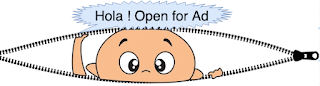## Latest Posts

### Control flow in python:-

Python supports various decision making contrl structures like If-elif-else, While /For Loops.
The colon character is used to delimit the beginning of a block of code and python uses concept of indented block to group block statements. In other words, python relies on the indentation
level to designate the extent of that block of code, or any nested blocks of code within. It is common for all control structures in python along with body of function and class.

What is indented block ?
: Below code lines print("I am Indented for if a==2") and print("All the best") are after a tab space(highlighted in yellow) with respect to if a==2 so it is termed as indented, however print("I am not Indented for if a==2") is at same level with respect to if a==2
if a==2:
print("I am Indented for if a==2")
print("all the best")
print("I am not Indented for if a==2")
Conditional statement - if/elif:- Write a sample code to check whether input number is divisible by 2/divisible by 5 or some other case.
input_number = 28   # input is hard coded
if input_number% 2 ==0: # Please note :(Colon here)
print "Even number, divisible by 2"
elif input_number% 5== 0 and input_number% 2 != 0:
print "Divisible by 5 but odd number"
else:
print "It is some thing different case"
Here pay attention to colon after each conditional statement and indentation after each of that.It is beauty of python which abstain developer from putting opening and closing braces(and avoid verbosity).

While loop: Write a sample code to print 1 to 10. Here J is initialized to 1 and on each iteration j is compared with 1, if it is less it prints value of j and increment j.
j = 1
while j < 11: # Please note :(Colon here)
print j
j= j+1
For loop:- Python's For loop is mainly used for iterating through series of elements like List, set, dict, etc. Consider the syntax of for loop for the time being, we will use For loop in detail when we learn these data structures.
for element in <iterable>:  #<Iterable> = i in range(0,5) or xrange(6) , etc
body
Python also support Break and Continue Statements. As all programming suggest to use these two control structures sparingly.
Python does not support switch/case as found in other languages. However we can simulate the same using dictionary and separate method call

```# define the function blocks
def zero():
print "You typed zero.\n"

def sqr():
print "n is a perfect square\n"

def even():
print "n is an even number\n"

def prime():
print "n is a prime number\n"

# map the inputs to the function blocks
options = {0 : zero,
1 : sqr,
4 : sqr,
9 : sqr,
2 : even,
3 : prime,
5 : prime,
7 : prime,
}

#Then the equivalent switch block is invoked:

options[num]()```

### Sample example using control statements:

1. Convert decimal to Binary.
```def decToBinary(n):
k = [];
x= n
while (n>0):
a=int(float(n%2))
k.append(a)
n=(n-a)/2
string=""
for j in k[::-1]:
string=string+str(j)
print('The binary no. for %d is %s'%(x, string))```
2. Find factorial of a number
```def factorial (num):
if(num==0):
return 1;
else:
return num*factorial (num-1)
n = int(raw_input().strip())
CutCount = factorial (n)
print CutCount
```
3. Check even and odd number, read input from stdin
```def evenCall(str1):
s = ""
for i in range(0,str1.__len__()):
if i%2 == 0:
s+=str1[i]
return s

def oddCall(str1):
s = ""
for i in range(0,str1.__len__()):
if i%2 != 0:
s+=str1[i]
return s
#Read number on input to be tested
input = int(raw_input())
for i in range(0,input):
1.